# Socle

(diff) ← Older revision | Latest revision (diff) | Newer revision → (diff)

of a module

The sum of all its simple submodules. When there are none, the socle is taken to be 0. In accordance with this definition one can consider in a ring its left and right socle. Each of them turns out to be a two-sided ideal that is invariant under all endomorphisms of the ring. The socle can be represented as a direct sum of simple modules. Completely-reducible modules (semi-simple modules) can be characterized as modules that coincide with their socle.

A submoduleof a moduleis large, or essential, if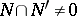for every non-zero submoduleof. A complement (respectively, essential complement) ofinis a submodule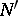such thatand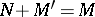(respectively,and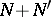is large). A module is complemented if each submodule has a complement. Each submodule always has a (not necessarily unique) essential complement. A module is complemented if and only if it is completely reducible and hence if and only if it coincides with its socle. The socle ofcan also be defined as the intersection of all the essential submodules of. The socle is the largest semi-simple submodule.
More generally, for a modular lattice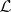an element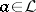is large or essential if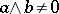for all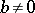. The socle of a modular lattice is defined as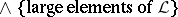. The interval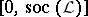is a complemented lattice.